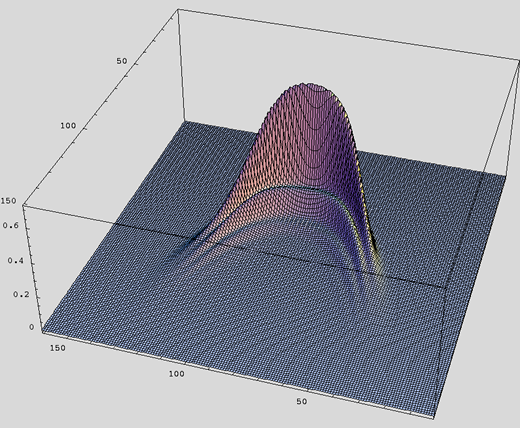## The H2 problem:

Simple problems are useful to test various approximation techniques. The simplest electron-molecule scattering problem should be scattering electrons off of hydrogen. We can solve the Schrodinger equation various ways and then test approximations against these solutions.

Unfortunately, the best solutions we have disagree with experiment by as much as 60%. That's just two protons and three electrons, folks, and we still can't get agreement with experiment.

The solution may lie in kinetic theory. Michael Morrison, Robert Robson and I have been looking at the assumptions of the analysis of the experiments. Toy models in which there is a steep increase in inelastic scattering at some large threshold produce non-Gaussian distributions (see figure below). All of the classical analysis assumed the electron swarm experiments were Gaussian. Could this the be the source of the discrepancy? We'll know soon!Above: Phase space distribution function for electrons with elastic and inelastic scattering when there is a large inelastic threshold. The horizonal axis is momentum (ranging from negative to positive). The axis forward from the page is position. The packet is drifting forward in time. Note the ridges in the distribution: they arise from the large inelastic threshold.